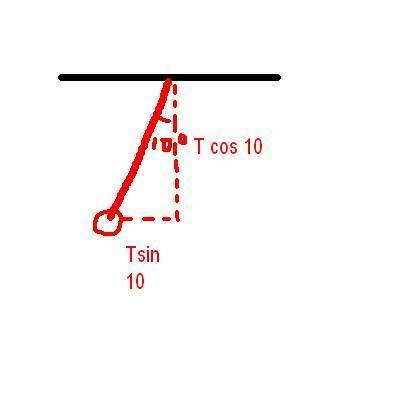# Pendulum in a Car: Solving Homework Statement

• phizics09
In summary, a pendulum of mass 1.0 kg suspended from the roof of a car traveling on a level road with an angle of 10 deg with the vertical indicates that the car is accelerating at a rate of mg tan10 deg divided by the radius of the pendulum. The equations used are Tcos10=mg and Tsin10=ma, which can be simplified to tan10= a/g. The mass of the pendulum does not affect the calculation as it cancels out in the equation.

## Homework Statement

A pendulum of mass 1.0 kg is suspended from the roof of a car traveling on a level road. An observer in the car notices that the pendulum string makes an angle of 10 deg with the vertical. What is the acceleration of the car?

Fc=mv^2/r

## The Attempt at a Solution

I drew the FBD diagram (attached) and I wrote the vertical and horizontal force equations:
Vertically: Tcos 10 deg=mg
Horizontally: Fc=mv^2/r=Tsin10deg.
So I got he equation mgtan10deg=mv^2/r after putting the equations together. But I don't know the radius of the pendulum, so I don't know how to solve the problem.

Thanks!Why do you use the centripetal force? Does anything move along a circle?

ehild

The suspended mass accelerates together with the car. Some force has to exert the force needed. It can be only the tension in the string, if it makes an angle with the vertical. The horizontal component of the tension is equal to ma (a is the common acceleration of the car and the pendulum) and the vertical component is equal and opposite to gravity, mg.

•simplyphysics
Oh i got it! Are the equations:
Tcos10=mg
Tsin10=ma

Sub T=mg/cos10 into Tsin10=ma, cancel out the m's and solve for a?

Actually,it gives you mass in the problem, so I don't know if I should cancel it out or not :S

As you get the a single equation for a from those two, the mass cancels by itself, but if you like to multiply and divide by m and making rounding errors, just do it:tongue:

ehild

I see, thanks :D

•simplyphysics